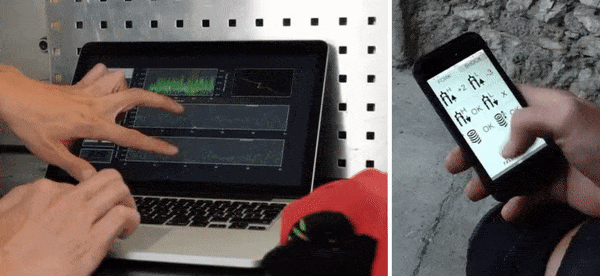WHAT IS DATA ACQUISITION?

"Data acquisition is the process of sampling signals that measure real-world physical conditions and converting the resulting samples into digital numeric values that can be manipulated by a computer. Data acquisition systems (DAQ), typically convert analog waveforms into digital values for processing."

The components of data acquisition systems include:

• Sensors, to convert physical parameters to electrical signals.

• Signal conditioning circuitry and analog-to-digital converters to convert conditioned sensor signals to digital values.WHY THE SAMPLING RATE IS CRUCIAL?

"A sample is a value or set of values at a point in time and/or space. A sampler is a subsystem or operation that extracts samples from a continuous signal.

The unit of measure is Hz (1/s)."The main idea is to reproduce the continuous signal as accurately as possible. In order to do this, it's important to relate the sampling frequency to the application area.

For low-speed phenomena (for example the variation of temperature in a room) a low sampling rate is enough, while high-speed ones will need a higher sampling rate.

The minimum sampling rate accepted in road bike applications is around 400Hz. So, being the speeds on MTB more than 5 times faster it's important to increase the sampling rate in order to get an accurate signal.

The figure above contains two different signals propers of a high-speed suspension displacing.

The red one is acquired at 300Hz, while the green one is acquired at 1000Hz. As you can see, the red signal is missing

part of the information that the green line shows.

This was one reason that pushed us to increase the sampling rate in BYB v2.0, now is 1000Hz for a [real] high-speed performance.

THE ELABORATION OF DATAThis is the final part of our work:

if everything was collected in the right way, we'll be able to give value at all of these points by using mathematical functions, statistical studies, and ad-hoc algorithms.

You'll be able to find the answers you are looking for through charts, metrics, statistical analysis, and comparisons.

Everything was ready for you directly inside our software and app.